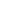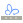# Routing, formulae and calculations

Cxoice Questionnaires and Scripts include full routing, branching logic, calculations and formulae with capabilities for text-piping, advanced filters and routing, and on-the-fly calculations. Formulas are available on all versions of Cxoice including the free HTML Questionnaire Editor and server-less questionnaires.

### Routing and skip patterns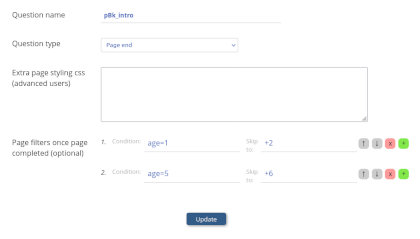All Cxoice, questionnaires and scripts come with options for page-by-page display, allowing multiple questions on the same page. The pagebreak question allows for skip patterns and calculations to be used on completion of the page.

These filters can include routing based on any previous question, or uploaded data from the sample and include calculations and filters.

In the pagebreak question the condition to be tested, and then which page the questionnaire should skip to. For instance age=1 and skip to page +2.

The skips can be to a specific page number, to a question by question name where the skip will be to the page the question, or a relative skip. For instance +2 would go 2 pages on and so skip the next page.

The routing also allows for error checking, but setting the skip to page to be 'error'. In which case if the condition is met, the participant will be shown an error message and asked to correct or rectify it before they continue.

### Reveal question

For more simple situations where one question leads to another being asked, an alternative option is a 'Reveal' question. In this case picking a particular answer reveals a hidden question on the same page. For instance, answering yes at 'Are you working?' might then show a hidden question about the type of job.

Cxoice has reveal questions for singles and dropdowns.

### Sections

Cxoice also allows for the use of 'Sections'. A section is a pop-up block of questions to be asked based on an answer given or due to randomisation. Sections are used for 'Flymenu' questions to ask follow up questions based on what the participant selected - ie a menu, and are part of what we call non-linear questionnaire design.

Sections can also be used in Table Questions, where a section is to be repeated like additional rows in a table. For instance, asking age and gender for each member of a household where the household size will vary.

And for advanced use, Sections can be used to randomise the order in which questions are asked under the control of the survey designer, where 'order effects' are thought to affect the answers given. For instance asking a follow up set of questions for a set of brands.

### Calculated variables

For calculations, Cxoice has three question types that can be used depending on whether the values are to be saved to the datafile or not. Calculations are always executed on the page in which they appear. Thus it's possible to take existing answers to create new variables for routing or error checking.

'Derived' makes a calculation and then saves the answer to the respondent data file. This can be used when selecting a test item at random, but where you want the same test to always be shown to the same respondent, and to know which test the respondent saw, for analysis.

'TempVar' is the same as 'Derived' but not saved. For instance, where intermediate calculations are done, or where the answer will not be used in analysis, for instance to make a complex formulae easy to write and read.

'List' is a list of items which can correspond to a master list that is used repeatedly, a list of items selected (derivedlist) that is then used for a follow up question. For instance, which of these brands have you heard of? Which brands have you seen advertising for recently?

### Text piping

Answers from previous questions can be 'piped' into later questions using %%GETVALUE[qname] or %%[qname]. Variables and calculations can be done by including the formula in the %%[...] brackets. For example %%[unitprice*volume] to show total expenditure.

### Formula in conditions and calculations

Any text item will be interpreted as a question name in a formula or a condition, or when used in the %%[...] calculation method. The value in the calculation will be the value of the answer given to the specific question.

For example, if 'age' is a write in or numeric, then 'age' will return the value written in.

However, where 'age' is a single or multiple, 'age' will return the code of the answer to a question with the name 'age'. So if 16-24 is code 1 and 16-24 is selected, 'age' will return 1.

To retrieve the code text instead of just the value use %%GETTEXT(age) or %%[GETTEXT(age)] which return the text string.

If you require a string - ie not a value based on a question name, then start the string with @. In other words @age returns the text 'age' rather than the value for age.

Basic conditions include =, <, <=, >, >=, !=, NOT (or !), OR (or ||), AND (or &&) and full value checks of == and !==.

Common functions for calculations include +,-, *, /, ^, %, LOG, EXP, SIN, COS, TAN, SQRT, DATEDIFF, ROUND, RANDOM and IF which can return a value if true or a value if false IF(AGE=1,@Under 24,@Over 24)

### Formulas for multiples

Multiple questions return a list of codes selected and consequently need handling a little differently to single or write-in type questions.

COUNT will give the number of answers to a multiple question (or 1 for a single). For instance if you want to find most preferred from a list a skip is needed if only one is given COUNT(brandsseen)=1 skip to +2

To check if a value has been selected in a multiple use HAS, IN (they're equivalent) eg use HAS(brandsseen,1) which will return true if the list of answers to brandsseen includes the value 1.

A similar function is MULTICHECK which will return true or false based on value of the code.

### Formulas for lists

List filtering is a common method of simplifying questionnaires automatically. For instance from 'Which brands have you heard of?', you would only want to follow up with the question 'Which brands have you seen advertising for recently?' for those brands heard of.

Lists can be manipulated using the GETLIST formula or GETGRIDLIST formula. In a formula a list can be built using commas eg 1,2,3

GETLIST(baselist, pickitems) will take a baselist (which could be a list question) and then apply the pickitems to get a new list. An options third parameter is exclude which inverts the selection.

For instance GETLIST(brands, brandsheardof) which can then be piped into the advertising seen follow up to ask 'Which of the brands you have heard of, have you seen advertising for recently?"

If %%GETLIST(...) is used as the first item in a codelist for a single or multiple question, then the list will be taken from the formula allowing lists to feed across questions.

GETGRIDLIST works in a similar fashion based on a specified column selected in a grid.

PICKITEM(list, code, alt) will get the value of the list for code if true, or alt if false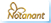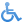Access level: public

Page feedback

This site uses essential cookies only. By continuing to use this site you accept our use of cookies: OK